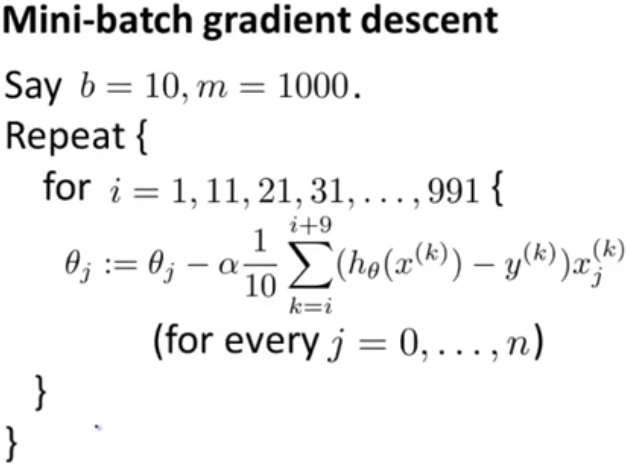signed

QiShunwang

“诚信为本、客户至上”

# Large scale machine learning - Mini-batch gradient descent

2021/3/21 7:55:56   来源：

In the previous class, we have talked about Stochastic gradient descent and how it can be faster than Batch gradient descent. In this class, let's talk about Mini-batch gradient descent. It can sometimes work even faster than Stochastic gradient descent.

To summarize:

• Batch gradient descent: use all m examples in each iteration
• Stochastic gradient descent: use 1 example in each iteration

The Mini-batch gradient descent is somewhere in between. Rather than using 1 example or m examples, we'll use b examples in each iteration, where b is called the "Mini-batch size". And typical value for b is 10; and typical range will be 2 ~ 100.figure-1

It shows the Mini-batch gradient descent algorithm in above figure-1. Here we have a batch size of 10 and 1000 training examples. So we perform this sort of gradient descent update using 10 examples at a time. And we need 100 steps of size 10 in order to get through all 1000 training examples.

Comparing to Batch gradient descent, this also allows us to make progress much faster. Again let's say we have 300,000,000 examples:

• With Batch gradient descent, we need scan through the entire training set before we can make any progress
• With Mini-batch gradient descent, after looking at just the first 10 examples, we can start to make progress in improving the parameters s. And then we can look at the second 10 examples and modify the parameters a little bit again.